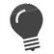# ASCII

Convert a decimal value into an ASCII character

 WTSupported in traditional Synergy on Windows WNSupported in Synergy .NET on Windows USupported on UNIX VSupported on OpenVMS
```xcall ASCII(decimal, ascii)
```

Arguments

decimal

An arithmetic expression whose value will be converted into a single ASCII character. (n)

ascii

A variable to be loaded with the ASCII character derived from the decimal value. (a)

Discussion

The ASCII subroutine converts a decimal value into a single ASCII character and stores it left-justified over blanks in a destination variable.

If the specified arithmetic expression yields a value greater than 255, Synergy DBL divides the value by 256 and uses the remainder to determine the ASCII character. For example, a value of 572 is interpreted as 60.

The ASCII character that is stored corresponds to the 8-bit binary pattern represented by the decimal value. For example, a value of 67 causes an uppercase letter “C” to be stored in the destination variable.

The character is stored in the leftmost position of the destination variable. The remaining character positions of the destination variable are cleared to blanks.%CHAR is more efficient than the ASCII subroutine for literal values, because %CHAR is evaluated at compile time.

Examples

The following program scans a stream file for a line feed embedded in a record and replaces it with a blank.

```record
data        ,a30
i           ,i4
ascii       ,a1
loc         ,d2

proc
xcall ascii(10, ascii)
repeat
begin
incr i
gets(2, data, i) [eof=eof]
if (loc = %instr(1, data, ascii))
begin
clear data(loc:1)
put(2, data, i)
end
end
close 2
stop
end
```